Home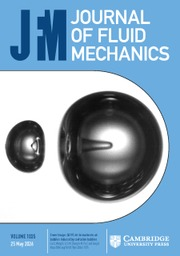Journal of Fluid Mechanics

# Universal fluctuations in the bulk of Rayleigh–Bénard turbulence

Published online by Cambridge University Press:  06 September 2019

Corresponding

## Abstract

We present an investigation of the root-mean-square (r.m.s.) temperature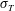$\unicode[STIX]{x1D70E}_{T}$ and the r.m.s. velocity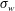$\unicode[STIX]{x1D70E}_{w}$ in the bulk of Rayleigh–Bénard turbulence, using new experimental data from the current study and experimental and numerical data from previous studies. We find that, once scaled by the convective temperature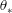$\unicode[STIX]{x1D703}_{\ast }$, the value of$\unicode[STIX]{x1D70E}_{T}$ at the cell centre is a constant (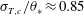$\unicode[STIX]{x1D70E}_{T,c}/\unicode[STIX]{x1D703}_{\ast }\approx 0.85$) over a wide range of the Rayleigh number (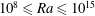$10^{8}\leqslant Ra\leqslant 10^{15}$) and the Prandtl number (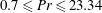$0.7\leqslant Pr\leqslant 23.34$), and is independent of the surface topographies of the top and bottom plates of the convection cell. A constant close to unity suggests that$\unicode[STIX]{x1D703}_{\ast }$ is a proper measure of the temperature fluctuation in the core region. On the other hand,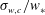$\unicode[STIX]{x1D70E}_{w,c}/w_{\ast }$, the vertical r.m.s. velocity at the cell centre scaled by the convective velocity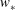$w_{\ast }$, shows a weak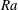$Ra$-dependence (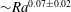${\sim}Ra^{0.07\pm 0.02}$) over$10^{8}\leqslant Ra\leqslant 10^{10}$ at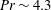$Pr\sim 4.3$ and is independent of plate topography. Similar to a previous finding by He & Xia (Phys. Rev. Lett., vol. 122, 2019, 014503), we find that the r.m.s. temperature profile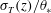$\unicode[STIX]{x1D70E}_{T}(z)/\unicode[STIX]{x1D703}_{\ast }$ in the region of the mixing zone with a mean horizontal shear exhibits a power-law dependence on the distance$z$ from the plate, but now the universal profile applies to both smooth and rough surface topographies and over a wider range of$Ra$. The vertical r.m.s. velocity profile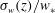$\unicode[STIX]{x1D70E}_{w}(z)/w_{\ast }$ obeys a logarithmic dependence on$z$. The study thus demonstrates that the typical scales for the temperature and the velocity are the convective temperature$\unicode[STIX]{x1D703}_{\ast }$ and the convective velocity$w_{\ast }$, respectively. Finally, we note that$\unicode[STIX]{x1D703}_{\ast }$ may be utilised to study the flow regime transitions in ultrahigh-$Ra$-number turbulent convection.

## JFM classification

Type
JFM Rapids
Information
Journal of Fluid Mechanics , 10 November 2019 , R1

## Access options

Get access to the full version of this content by using one of the access options below.

## References

Adrian, R. J. 1996 Variation of temperature and velocity fluctuations in turbulent thermal convection over horizontal surfaces. Intl J. Heat Mass Transfer 39, 23032310.CrossRefGoogle Scholar
Ahlers, G., Bodenschatz, E., Funfschilling, D., Grossmann, S., He, X., Lohse, D., Stevens, R. J. A. M. & Verzicco, R. 2012 Logarithmic temperature profiles in turbulent Rayleigh–Bénard convection. Phys. Rev. Lett. 109, 114501.CrossRefGoogle ScholarPubMed
Ahlers, G., Grossmann, S. & Lohse, D. 2009 Heat transfer and large scale dynamics in turbulent Rayleigh–Bénard convection. Rev. Mod. Phys. 81, 503537.CrossRefGoogle Scholar
Castaing, B., Gunaratne, G., Heslot, F., Kadanoff, L., Libchaber, A., Thomae, S., Wu, X.-Z., Zaleski, S. & Zanetti, G. 1989 Scaling of hard thermal turbulence in Rayleigh–Bénard convection. J. Fluid Mech. 204, 130.CrossRefGoogle Scholar
Chillà, F. & Schumacher, J. 2012 New perspectives in turbulent Rayleigh–Bénard convection. Eur. Phys. J. E 35, 58.Google ScholarPubMed
Daya, Z. A. & Ecke, R. E. 2001 Does turbulent convection feel the shape of the container? Phys. Rev. Lett. 87, 184501.CrossRefGoogle Scholar
Deardorff, J. W. 1970 Convective velocity and temperature scales for the unstable planetary boundary layer and for Rayleigh convection. J. Atmos. Sci. 27, 12111213.2.0.CO;2>CrossRefGoogle Scholar
Du, Y. B. & Tong, P. 2000 Turbulent thermal convection in a cell with ordered rough boundaries. J. Fluid Mech. 407, 5784.CrossRefGoogle Scholar
Du, Y. B. & Tong, P. 2001 Temperature fluctuations in a convection cell with rough upper and lower surfaces. Phys. Rev. E 63, 046303.Google Scholar
Grossmann, S. & Lohse, D. 2004 Fluctuations in turbulent Rayleigh–Bénard convection: the role of plumes. Phys. Fluids 16, 44624472.CrossRefGoogle Scholar
He, X., Funfschilling, D., Nobach, H., Bodenschatz, E. & Ahlers, G. 2012 Transition to the ultimate state of turbulent Rayleigh–Bénard convection. Phys. Rev. Lett. 108, 024502.CrossRefGoogle ScholarPubMed
He, Y.-H. & Xia, K.-Q. 2019 Temperature fluctuation profiles in turbulent thermal convection: a logarithmic dependence versus a power-law dependence. Phys. Rev. Lett. 122, 014503.CrossRefGoogle ScholarPubMed
Heslot, F., Castaing, B. & Libchaber, A. 1987 Transitions to turbulence in helium gas. Phys. Rev. A 36, 58705873.CrossRefGoogle ScholarPubMed
Kaczorowski, M., Chong, K.-L. & Xia, K.-Q. 2014 Turbulent flow in the bulk of Rayleigh–Bénard convection: aspect-ratio dependence of the small-scale properties. J. Fluid Mech. 747, 73102.CrossRefGoogle Scholar
Lakkaraju, R., Stevens, R. J. A. M., Verzicco, R., Grossmann, S., Prosperetti, A., Sun, C. & Lohse, D. 2012 Spatial distribution of heat flux and fluctuations in turbulent Rayleigh–Bénard convection. Phys. Rev. E 86, 056315.Google ScholarPubMed
Lohse, D. & Xia, K.-Q. 2010 Small-scale properties of turbulent Rayleigh–Bénard convection. Annu. Rev. Fluid Mech. 42, 335364.CrossRefGoogle Scholar
Lui, S.-L. & Xia, K.-Q. 1998 Spatial structure of the thermal boundary layer in turbulent convection. Phys. Rev. E 57, 54945503.Google Scholar
Niemela, J. J., Skrbek, L., Sreenivasan, K. R. & Donnelly, R. J. 2000 Turbulent convection at very high Rayleigh numbers. Nature 404, 837840.CrossRefGoogle ScholarPubMed
Qiu, X. L., Shang, X.-D., Tong, P. & Xia, K.-Q. 2004 Velocity oscillations in turbulent Rayleigh–Bénard convection. Phys. Fluids 16, 412423.CrossRefGoogle Scholar
Scheel, J. D. & Schumacher, J. 2016 Global and local statistics in turbulent convection at low Prandtl numbers. J. Fluid Mech. 802, 147173.CrossRefGoogle Scholar
Shang, X.-D., Tong, P. & Xia, K.-Q. 2008 Scaling of the local convective heat flux in turbulent Rayleigh–Bénard convection. Phys. Rev. Lett. 100, 244503.CrossRefGoogle ScholarPubMed
Shen, Y., Xia, K.-Q. & Tong, P. 1995 Measured local-velocity fluctuations in turbulent convection. Phys. Rev. Lett. 75, 437440.CrossRefGoogle ScholarPubMed
Sun, C., Cheung, Y.-H. & Xia, K.-Q. 2008 Experimental studies of the viscous boundary layer properties in turbulent Rayleigh–Bénard convection. J. Fluid Mech. 605, 79113.CrossRefGoogle Scholar
Wang, J. & Xia, K.-Q. 2003 Spatial variations of the mean and statistical quantities in the thermal boundary layers of turbulent convection. Eur. Phys. J. B 32, 127136.CrossRefGoogle Scholar
Wang, Y., Xu, W., He, X., Yik, H., Wang, X., Schumacher, J. & Tong, P. 2018 Boundary layer fluctuations in turbulent Rayleigh–Bénard convection. J. Fluid Mech. 840, 408431.CrossRefGoogle Scholar
Wei, P. & Ahlers, G. 2016 On the nature of fluctuations in turbulent Rayleigh–Bénard convection at large Prandtl numbers. J. Fluid Mech. 802, 203244.CrossRefGoogle Scholar
Wei, P., Chan, T.-S., Ni, R., Zhao, X.-Z. & Xia, K.-Q. 2014 Heat transport properties of plates with smooth and rough surfaces in turbulent thermal convection. J. Fluid Mech. 740, 2846.CrossRefGoogle Scholar
Xia, K.-Q. 2013 Current trends and future directions in turbulent thermal convection. Theor. Appl. Mech. Lett. 3, 052001.CrossRefGoogle Scholar
Xia, K.-Q., Lam, S. & Zhou, S.-Q. 2002 Heat-flux measurement in high-Prandtl-number turbulent Rayleigh–Bénard convection. Phys. Rev. Lett. 88, 064501.CrossRefGoogle ScholarPubMed
Xie, Y.-C., Ding, G.-Y. & Xia, K.-Q. 2018 Flow topology transition via global bifurcation in thermally driven turbulence. Phys. Rev. Lett. 120, 214501.CrossRefGoogle ScholarPubMed
Xie, Y.-C. & Xia, K.-Q. 2017 Turbulent thermal convection over rough plates with varying roughness geometries. J. Fluid Mech. 825, 573599.CrossRefGoogle Scholar

### Full text views

Full text views reflects PDF downloads, PDFs sent to Google Drive, Dropbox and Kindle and HTML full text views.

Total number of HTML views: 23
Total number of PDF views: 457 *
View data table for this chart

* Views captured on Cambridge Core between 06th September 2019 - 18th January 2021. This data will be updated every 24 hours.

Hostname: page-component-77fc7d77f9-n279q Total loading time: 0.288 Render date: 2021-01-18T21:19:46.379Z Query parameters: { "hasAccess": "0", "openAccess": "0", "isLogged": "0", "lang": "en" } Feature Flags last update: Mon Jan 18 2021 20:55:55 GMT+0000 (Coordinated Universal Time) Feature Flags: { "metrics": true, "metricsAbstractViews": false, "peerReview": true, "crossMark": true, "comments": true, "relatedCommentaries": true, "subject": true, "clr": true, "languageSwitch": true, "figures": false, "newCiteModal": false, "shouldUseShareProductTool": true, "shouldUseHypothesis": true, "isUnsiloEnabled": true }

# Send article to Kindle

Note you can select to send to either the @free.kindle.com or @kindle.com variations. ‘@free.kindle.com’ emails are free but can only be sent to your device when it is connected to wi-fi. ‘@kindle.com’ emails can be delivered even when you are not connected to wi-fi, but note that service fees apply.

Find out more about the Kindle Personal Document Service.

Universal fluctuations in the bulk of Rayleigh–Bénard turbulence
Available formats
×

# Send article to Dropbox

To send this article to your Dropbox account, please select one or more formats and confirm that you agree to abide by our usage policies. If this is the first time you use this feature, you will be asked to authorise Cambridge Core to connect with your <service> account. Find out more about sending content to Dropbox.

Universal fluctuations in the bulk of Rayleigh–Bénard turbulence
Available formats
×

# Send article to Google Drive

To send this article to your Google Drive account, please select one or more formats and confirm that you agree to abide by our usage policies. If this is the first time you use this feature, you will be asked to authorise Cambridge Core to connect with your <service> account. Find out more about sending content to Google Drive.

Universal fluctuations in the bulk of Rayleigh–Bénard turbulence
Available formats
×
×